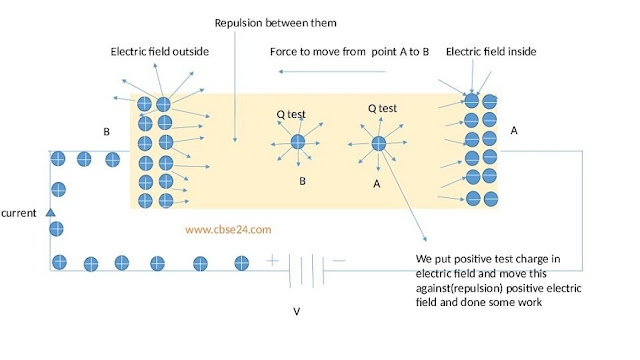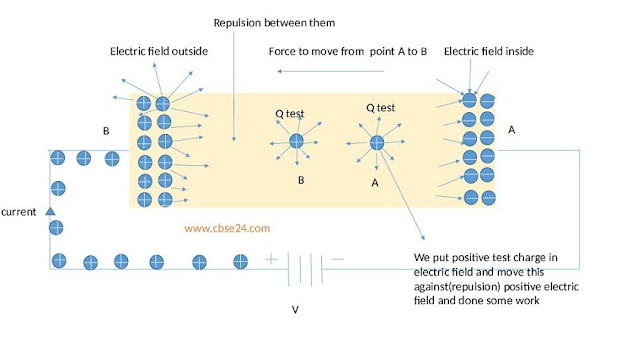# Electrical Potential and potential difference class 10 | cbse24.com

## Electrical Potential

When a small positive test charge is placed in the electric field due to another charge, it experiences a force. So, work has to be done on the positive test charge to move it against this force of repulsion (we move the test charge from point A to point B, so some work is done occurs). The electric potential at a point in an electric field is defined as the work in moving a unit test charge from infinity to that point.

Conclusion-

Potential is denoted by the symbol V and its unit is volt. A potential of 1 volt at a point means that 1 joule of work is done in moving 1 unit positive charge from infinity to that point.

Concept to Understand-

1. We first take a positive test charge Q test at point A which field is outward and another charge also outward field so Q test feels repulsion if move this test charge against another positive charge.

2. Suppose this Q test is at infinity (anywhere in that field) and move this test charge to a particular point B, so some work is done to perform by this test charge to move from point A to point B against the electric field.

Vab=Wab/Qtest(+ve)

so, if suppose test charge at infinity

than Vb-V∞ = Wb-W∞/Qtest(+ve)

so V∞=0 and W∞=0 putting this value

so electric Potential at point B is

Vb=Wb/Qtest(+ve)

V at b=1volt mean W at b=1joule to bring Qtest =1 coulomb at point B from infinityElectric Potential

## Potential Difference

The difference in electric potential between two points is known as potential difference. The potential difference between two points in an electric circuit is defined as the amount of work done in moving a unit charge from one point to the other point.
So that.

Work done
P.d(V) =    --------------------
Quantity of charge moved

W
V =    ---------
Q net

• The SI unit of potential difference is ----Volt
• It is denoted by symbol ---V
• The potential difference is measured by the mean of an instrument called ------Voltmeter.
• The voltmeter is another name for potential difference (p.d.)
• It is connected in parallel in the circuit to measure voltageVoltmeter connection to measure voltage

### A mixed question of S.Chand and NCERT of this topic.

Q1. How much energy is given to each coulomb of charge passing through a 6V battery?

Ans. V=W/Q

6=W/1C

W=6X1=6 joule

energy given to each coulomb of charge is 6 joules.

Q2. Three 2 V cells are connected in series and used as a battery in a circuit.

(a) What is p.d at the terminals of the battery?

(b) How many joules of electrical energy does 1C gain on passing through (i)one cell (ii)all three cells?

Ans. (a) P.d. at the terminal of the battery=2v+2v+2v=6V

(b) (i) electrical energy through 1 cell =VxQ=2Vx1C=2J

(ii) Electrical energy through 3 cell =nxVxQ=3x2x1c=6J

(n=no of the cell)

"Test your knowledge by giving online Exam(quiz)"

1. Test1 (5 quizzes each of 2 marks)*